# Micromass' big simulation challenge

• Challenge
Staff Emeritus
Homework Helper
Let's do some simulations. Below are 5 simulation problems that require a computer to solve. Use any language you want. Post the answers (including graphics) and code here!

• Any use of outside sources is allowed, but do not look up the question directly. For example, it is ok to go check probability books, but it is not allowed to google the exact question.
• All programming languages are allowed.
• Please reference every source you use.

1. SOLVED BY Ibix A clinic has three doctors. Patients come into the clinic at random, starting at 9a.m., according to a Poisson process with time parameter 10 minutes: that is, the time after opening at which the first patient appea follows an exponential distribution with expectation 10 minutes and then, after each patient arrices the waiting time until the next patient is indepdnently exponentially distributed, also with expectation 10 minutes. When a patient arrices, he or she waits until a doctor is available. The amount of time spent by each doctor with each patient is a random variable, uniformly distributed between 5 and 20 minutes. The office stops admitting new patients at 4m and closes when the last patient is with the doctor.

Find the median and 50% interval for the following: the amount of patients coming to the office, the amount of patients having to wait, the average wait, the closing time of the office. Graph the distributions.

2. SOLVED BY mfb Write a program to simulate data from the standard normal distribution. The ONLY randomization program you can use is a program which automatically tosses a coin and gives you head or tails. Throughout the program, you cannot know what the probability of heads is (only that it is not 0 or 1). Provide proof that your program simulates the normal distribution.

3. SOLVED BY I_am_learning, Ygggdrasil Simulate the distribution from Challenge 5 of the probability challenge:
In a town of 1001 inhabitants, a person tells a rumor to two distinct people, the "first generation". These repeat the performance and generally, each person tells a rumor to two people at random without regard to the past development (a rumor cannot be told to the person who told him this rumor). Find the probability ##P(r)## that the generation ##1,2,...,r## will not contain the person who started the rumor.

4. SOLVED BY I_am_learning Repeat (3) but now suppoe that a person can tell the rumor to the person who told him the rumor. Do the result of 3 change significantly?

5. SOLVED BY Ibix The newsstand buys papers for 33 dollars each and sells them for 50 dollars each. Newspaper not sold at the end of the day are sold as scrap for 5 dollars each. Newspaper can be purchased in bundles of 10. thus the newsstand can buy 50, 60, and so on. There are three types of newsdays: ‘good’, ‘fair’ and ‘poor’ having probabilities 0.35, 0.45 and 0.20, respectively. The distribution of newspapers demanded on each of these days is given below in the table. Problem: Compute the optimal number of papers the newsstand should purchase.
$$\begin{array}{||c||c|c|c||} \hline \hline \text{Demand} & \text{Good day} & \text{Fair day} & \text{Poor day}\\ \hline \hline 40 & 0.03 & 0.1 & 0.44\\ \hline 50 & 0.05 & 0.18 & 0.22\\ \hline 60 & 0.15 & 0.4 & 0.16\\ \hline 70 & 0.2 & 0.2 & 0.12\\ \hline 80 & 0.35 & 0.08 & 0.06\\ \hline 90 & 0.15 & 0.04 & 0\\ \hline 100 & 0.07 & 0 & 0\\ \hline \hline \end{array}$$

Thank you all for participating! I hope many of you have fun with this! Don't hesitate to post any feedback in the thread!

1. Gelman et al "Bayesian Data Analysis"
2. Invented myself
3. Feller "An introduction to probability theory and its applications Vol1" Chapter II "Elements of Combinatorial analysis"
4. Feller "An introduction to probability theory and its applications Vol1" Chapter II "Elements of Combinatorial analysis"
5. http://www.slideshare.net/praveshnegi/chp-2-simulation-examples

Last edited:
•mheslep and Greg Bernhardt

CynicusRex
Gold Member
That rumor will haunt us forever; just like the ring.

Challenge 3 and 4:
Python:
__author__ = "I_am_learning"

import random

def rumorReturns(N,r): #returns the generation when the rumor returns back to the original person, otherwise returns -1 if it doesn't return
peoples = list(range(0, N, 1)) #Index is person, value is that person's source of rumor
first_man_index = random.randrange(0,N) #Index of first man
peoples[first_man_index]=first_man_index #the source of first person is himself

current_gen = [first_man_index]
generation = 1
while (generation<=r):
new_gen = []
for person in current_gen: #each person in the current gen will spread the rumor
while(True):
new_men = random.sample(range(N),2) #spread rumor to two random person

#Use this for problem 3
if new_men not in [person, peoples[person]] and new_men not in [person, peoples[person]]: #repeat until non of them is the current person or his source

#Use this for problem 4
#if new_men not in [person] and new_men not in [person]: #repeat until non of them is the current person; you can't rumor yourself
break
new_gen += new_men #add these new persons into new_generation bucket
peoples[new_men] = person #mark this person as the source of rumor for both
peoples[new_men] = person #mark this person as the source of rumor
if first_man_index in new_men:
#print("Got back the the first man")
return generation
generation += 1
current_gen = new_gen

return -1

Repeat = 10000 #How many times to repeat the experiment; larger number gives better answer,
ans = 0
for i in range(Repeat):
ans += 1 if rumorReturns(N=1001,r=5) > 0 else 0

print("The probability that the rumor returns back to the original source is: {0}".format(ans/Repeat))

For N=1001, and r=5
Problem 3: 0.0564
Problem 4: 0.0571
So, there appears to be a slight increase in the probability.

Last edited:
Staff Emeritus
Homework Helper
Can you perhaps provide some kind of histogram for different numbers of ##r## for both questions?

Staff Emeritus
Homework Helper
I'm not used to the specifics of the code, but to be sure, in the following line:

new_men =random.sample(range(N),2)#spread rumor to two random person

Are you sure you're picking two different elements here?

I'm not used to the specifics of the code, but to be sure, in the following line:

new_men =random.sample(range(N),2)#spread rumor to two random person

Are you sure you're picking two different elements here?
Yes, the python random.sample library generates random samples without replacement (i.e. unique samples)

I have included the histogram as requested for problem 3 and problem 4. They look quite the same.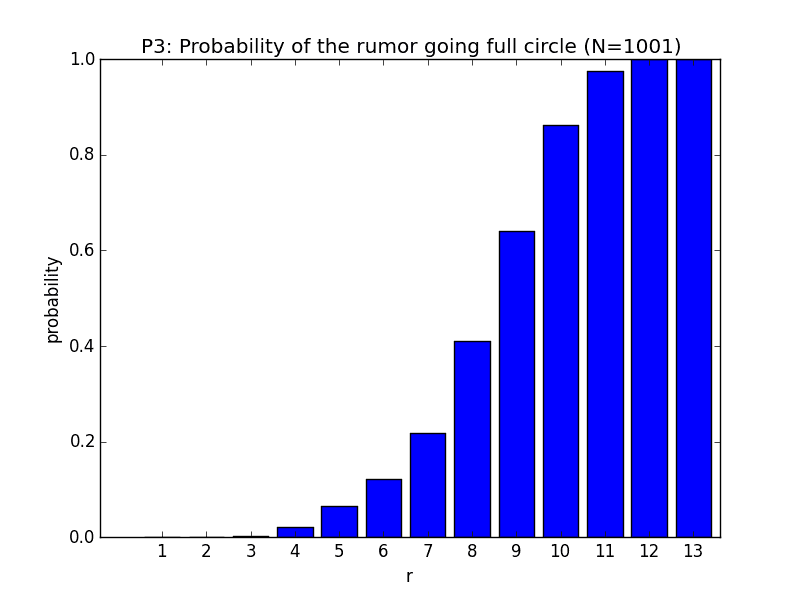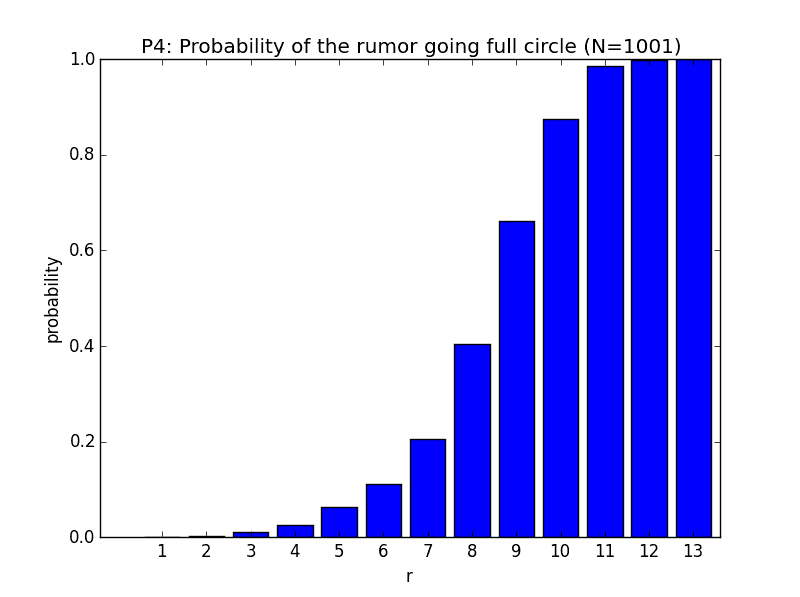•micromass
I realized I have a bug in my previous submission. When rumor is spread to next generation, there is a chance (which grows higher as generation increases) that the same person will be counted multiple times as a next gen candidate to spread rumor (because he was randomly selected by multiple parents). I have fixed this bug by using a set (no repetition) to store candidates for next generation. Below is the updated code (including plotting part), and new histograms.This change limits the growth of rumor compared to previous buggy version.

Python:
__author__ = "Rajendra Adhikari"
from matplotlib import pyplot
import random
import numpy

def rumorReturns(N,r): #returns the genertion when the rumor returns back to the original person, otherwise returns -1 if it doesn't return
peoples = list(range(0, N, 1)) #Index is person, value is that person's source of rumor
first_man_index = random.randrange(0,N) #Index of first man
peoples[first_man_index]=first_man_index #the source of first person is himself

current_gen = set([first_man_index])
generation = 1
while (generation<=r):
new_gen = set()
for person in current_gen: #each person in the current gen will spread the rumor
while(True):
new_men = random.sample(range(N),2) #spread rumor to two random person

#Use this for problem 3
#if new_men not in [person, peoples[person]] and new_men not in [person, peoples[person]]: #repeat until non of them is the current person or his source

#Use this for problem 4
if new_men not in [person] and new_men not in [person]: #repeat until non of them is the current person; you can't rumor yourself
break
new_gen |= set(new_men) #union these new persons into new_generation bucket
peoples[new_men] = person #mark this person as the source of rumor for both
peoples[new_men] = person #mark this person as the source of rumor
if first_man_index in new_men:
#print("Got back the the first man")
return generation
generation += 1
current_gen = new_gen

return -1

Repeat = 1000 #How many times to repeat the experiment; larger number gives better answer,
final_r = 13

probability = []
label = []
for k in range(final_r):
r = k+1
label += [str(r)]
ans = 0
for i in range(Repeat):
ans += 1 if rumorReturns(N=1001,r=r) > 0 else 0
probability += [ans/Repeat]
print("The probability for r={0} is {1}".format(r,ans/Repeat))

rs = numpy.array(range(1,14))
pyplot.bar(rs,probability)
pyplot.xticks(rs+0.4,label)
pyplot.xlabel('r')
pyplot.ylabel('probability')
pyplot.title('P4: Probability of the rumor going full circle (N=1001)')

pyplot.show()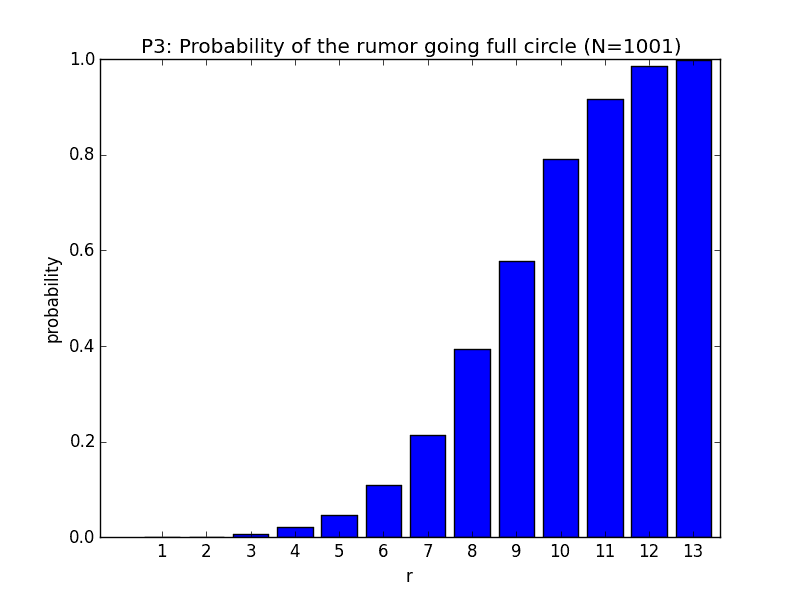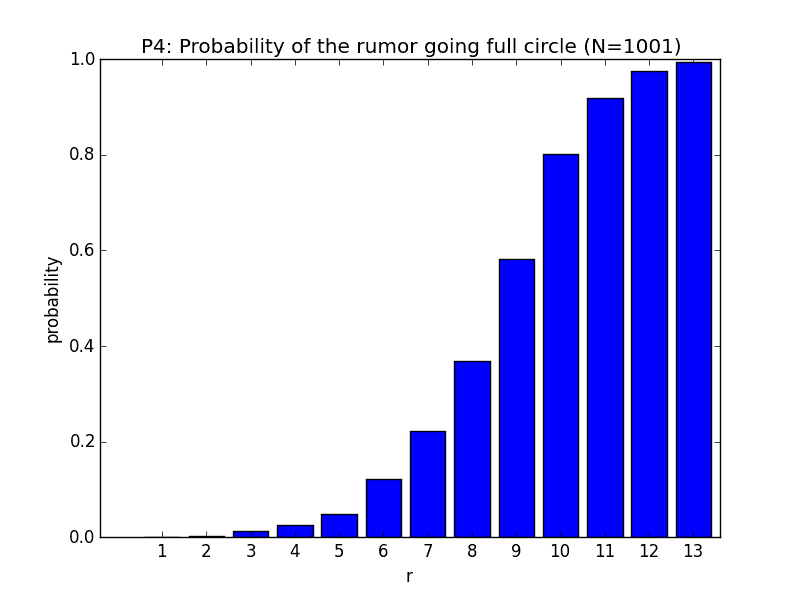Last edited:
Ygggdrasil
Gold Member
2021 Award
The graph I generate for #3 is quite different from the one above. For example, P(r) never reaches 1. Here's my semi-analytic, semi-numeric solution made while thinking of the answer to the probability challenge:

Building off of an approach similar to @mfb in post #91:

Let N(r) be the number of inhabitants in generation r. There are N(r) inhabitants who know the rumor and cannot pass it on to themselves, and n + 1 - N(r) inhabitants who do not currently know the rumor (let's call these inhabitants naive).

For case 2 (naive inhabitants), they have N(r) opportunities to hear the rumor in the next round. The probability that one rumor spreader passes the rumor to one particular individual is 2/n. Therefore, the probability that a naive individual does not hear the rumor is ##(1-2/n)^N##. The expected number of naive individuals to hear the rumor in the next generation is ##(n+1-N) (1 - p^N)##, where p = 1-2/n
.
For case 1 (rumor spreaders), they have N - 1 opportunities to hear the rumor as they cannot spread the rumor to themselves. Therefore, the probability that they hear the rumor during the next round is ##1 - p^{N-1}##, and the expected number of rumor spreaders who become part of generation r+1 is ##N(1-p^{N-1})##.

In total ##N(r+1) = (n+1-N) (1-p^N)+N (1-p^{N-1})## which simplifies to ##N(r+1) = (n+1) - (n+1 + \frac{2N}{n-2})p^N##

We can express, the fraction of inhabitants part of generation r, F(r), through the following recurrence relation: $$F(r+1) = 1-\left(1+\frac{2F(r)}{n-2}\right)\left(\frac{n-2}{n}\right)^{(n+1)F(r)}$$

Using the recurrence relation and F(1) = 2, I can plot F(r) for various values of n: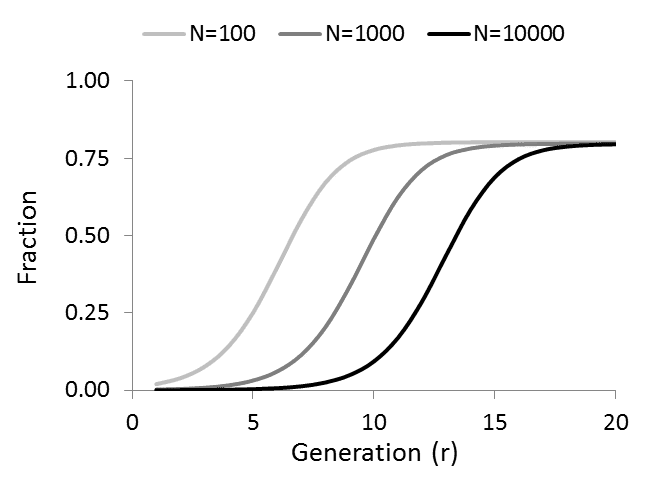Solving the recurrence relation analytically would give a solution to the probability challenge, but I'm not sure how to do that.

mfb
Mentor
• Write a program to simulate data from the standard normal distribution. The ONLY randomization program you can use is a program which automatically tosses a coin and gives you head or tails. Throughout the program, you cannot know what the probability of heads is (only that it is not 0 or 1). Provide proof that your program simulates the normal distribution.
If we don't care about efficiency, in C++ reduced to the relevant parts:
Code:
//here we get our coin flip, heads=true, tails=false
bool biasedcoinflip() {
...
}

//here we get a fair coin flip, independent of the bias of biasedcoinflip():
//we interpret "HT" as heads and "TH" as tails, otherwise we repeat.
bool faircoinflip() {
while(1) {
bool flip1=biasedcoinflip();
bool flip2=biasedcoinflip();
if( flip1 && !flip2 ) return true;
if( !flip1 && flip2 ) return false;
}
}

double getstandardnormvalue() {
double precision=0.01; //we could also leave that up to the user if we want
int maxN=1/(precision*precision);
double sum=0;
for(int n=0;n<maxN;n++) {
if(faircoinflip) sum++;
}
return (2*sum-maxN)/sqrt(maxN);
}
Proof: HT and TH have the same probability, assuming subsequent coin tosses are independent. The sum follows a binomial distribution which (for large maxN) approximates a Gaussian with mean maxN/2 and variance maxN/4. The numerator follows a Gaussian with mean 0 and variance maxN, the denominator scales it to a variance of 1. We get a step size of 0.01, which can be reduced arbitrarily to improve the precision.

The Gaussian part is horribly inefficient, and the HT/TH part is not optimal either, but the question didn't ask for the fastest solution.

•micromass
Staff Emeritus
Homework Helper
For example, P(r) never reaches 1.

Somehow I find that intuitively very unlikely...

Ygggdrasil
Gold Member
2021 Award
Somehow I find that intuitively very unlikely...
Even if everyone knows the rumor in round x, there will be some small fraction of inhabitants who by chance do not hear the rumor in round x+1.

Or is this a first passage problem, and you're asking about when the originator will first re-hear the rumor?

mfb
Mentor
I understood P(r) as probability that a given person gets told the rumor in this generation, independent of the history.
It doesn't surprise me. In generation r-1, we have at most all people telling the rumors to others, so of course we can never guarantee that a given person will hear the rumor.

Staff Emeritus
Homework Helper
Even if everyone knows the rumor in round x, there will be some small fraction of inhabitants who by chance do not hear the rumor in round x+1.

I agree but that was not what was asked:

Or is this a first passage problem, and you're asking about when the originator will first re-hear the rumor?

Correct!

Ygggdrasil
Gold Member
2021 Award
Correct!

Ah, ok. I misread the definition of P(r) in this thread. P(r) was defined differently in the probability challenge thread which was the source of confusion.

Staff Emeritus
Homework Helper
Ah, ok. I misread the definition of P(r) in this thread. P(r) was defined differently in the probability challenge thread which was the source of confusion.

Your solution was very interesting though, even though it didn't answer the question. It showed that the later generations would all be about 3/4th of the maximal size. I'll credit you as well for this fun piece of insight!

Ibix
So I had a crack at #1, the doctors' office. Here's the code:
Python:
from __future__ import division

import math,random

# Constants
MEANTIME=10  # Mean time between arrivals
CLOSINGTIME=60*7  # Number of minutes surgery is open in a day
MINCONSULT=5  # Minimum consultation time
CONSULTRANGE=15  # Range of consultation times (=max time-MINCONSULT)

ITERATIONS=10000  # Number of loops round the simulation
DISPLAYSTEP=50  # Number of loops between printed iteration numbers
OUTFILE="C:\\Projects\\Physics\\Micromass simulation 1\\histos.dat"

# Empty arrays to hold results from each run of the sims
patientCount=[]  # the amount of patients coming to the office
waitCount=[]  # the amount of patients having to wait
averageWait=[]  # the average wait
closingTime=[]  # the closing time of the office.

# Simulation!
for i in xrange(ITERATIONS):
if (i+1)//DISPLAYSTEP*DISPLAYSTEP==i+1: print "Iteration: "+str(i+1)
# Initialise the first time the docs are free to zero minutes past 9am,
# the latest arrival to zero minutes past 9am, and the number of patients
# to zero
docsBecomeFree=[0,0,0]
lastArrival=0
patients=0
waited=0
totalWait=0
while True:
# Generate an arrival - but if they arrived after closing time,
# terminate the sim and they're out of luck
lastArrival-=MEANTIME*math.log(random.random())
if lastArrival>CLOSINGTIME:
break
patients+=1
# Find when the next doc is free and calculate the waiting time for
# this patient
nextDocFree=min(docsBecomeFree)
waitTime=max(0,nextDocFree-lastArrival)
totalWait+=waitTime
# Find which doc was first available and make them busy to the end of
# this patient's consulting time
for doc in range(len(docsBecomeFree)):
if docsBecomeFree[doc]==nextDocFree:
consultTime=MINCONSULT+random.random()*CONSULTRANGE
if waitTime>0:
waited+=1
docsBecomeFree[doc]=nextDocFree+consultTime
else:
docsBecomeFree[doc]=lastArrival+consultTime
break
# Day has ended - add the MI to the logs
patientCount.append(patients)
waitCount.append(waited)
averageWait.append(totalWait/patients)
closingTime.append(max(docsBecomeFree+[CLOSINGTIME])-CLOSINGTIME)

# Analysis of results
def round2(val):
# Round the value to 2dp
return int(val*100)/100
def analyseArray(outfile,title,data,binWidth):
# Sort the array into increasing order
data.sort()
# Get the quartiles - this is only exact if len(data) is a multiple of 4,
# but the definition is ambiguous in other cases anyway.
firstQuartile=data[len(data)//4]
median=data[len(data)//2]
thirdQuartile=data[3*len(data)//4]
print title+" ("+str(len(data))+" iterations)"
print "Median: "+str(round2(median))
print "50% interval: "+str(round2(firstQuartile))+" -- "+str(round2(thirdQuartile))
print
# Determine the histogram
minVal=data
nBins=1+int((data[-1]-data)/binWidth)
histo=[0 for i in range(nBins)]
for d in data:
bin=min(nBins-1,int((d-minVal)/binWidth))
histo[bin]+=1
# Dump the histogram to the file
print >> outfile,"Bin\t"+title
for bin in range(nBins):
binEdge=bin*binWidth+minVal
print >> outfile,str(round2(binEdge))+"\t"+str(100*histo[bin]/len(data))
print >> outfile,"\n"

fp=file(OUTFILE,"w")
analyseArray(fp,"Amount of patients coming to the office",patientCount,1)
analyseArray(fp,"Amount of patients having to wait",waitCount,1)
analyseArray(fp,"Average wait",averageWait,0.25)
analyseArray(fp,"Closing time of the office",closingTime,1)
fp.close()
Basically it keeps track of the last arrival, each time adding ##-10\ln r## minutes, where ##r\sim U(0,1)##. It also keeps track of when the three doctors are finished with their current patient queue, and adds this patient on to the end of the first to become free, with a consultation time of c, ##c\sim U(5,20)##. I've taken "50% interval" to mean the range from the 25th to 75th percentile. The results I got are:

Amount of patients coming to the office (10000 iterations)
Median: 42.0
50% interval: 37.0 -- 46.0

Amount of patients having to wait (10000 iterations)
Median: 5.0
50% interval: 3.0 -- 9.0

Average wait (minutes - 10000 iterations)
Median: 0.46
50% interval: 0.21 -- 0.86

Closing time of the office (minutes after 4pm - 10000 iterations)
Median: 5.96
50% interval: 0.0 -- 11.2

Histograms: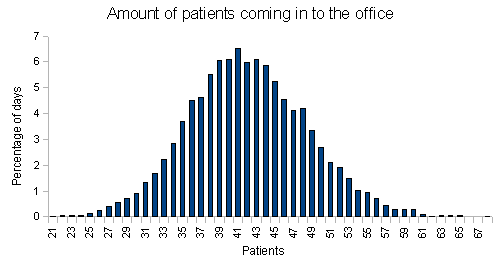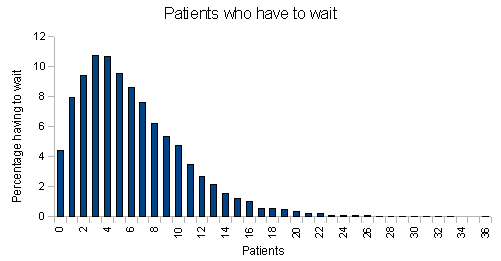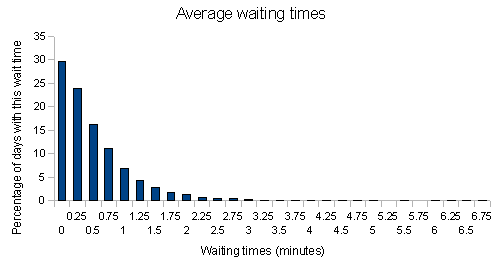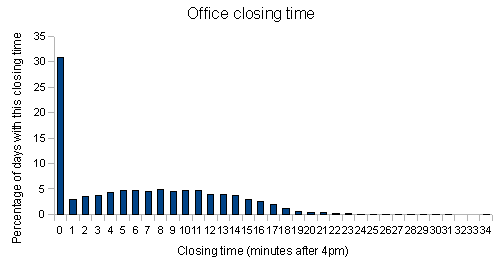Last edited:
•micromass
mfb
Mentor
:)

Does the news stand in problem (5) know in advance if a day is Good, Fair or Poor? If no, where is the point in making three groups, if yes, is it just the same problem with 3 different numbers?

Staff Emeritus
Homework Helper
Does the news stand in problem (5) know in advance if a day is Good, Fair or Poor?

No

Chestermiller
Mentor
No
Problem 5 looks like a standard Decision and Risk problem, which is usually solved by Monte Carlo simulation. Is the use of Monte Carlo allowed?

Staff Emeritus
Homework Helper
Problem 5 looks like a standard Decision and Risk problem, which is usually solved by Monte Carlo simulation. Is the use of Monte Carlo allowed?

Yep, as long as it's a simulation!

Ibix
Problem 5 can be done with pen and paper (unless I'm missing something) - old school computing. Is that cheating, or shall I post a photo?

I make it 60

Last edited:
•micromass
Chestermiller
Mentor
Problem 5 can be done with pen and paper (unless I'm missing something) - old school computing. Is that cheating, or shall I post a photo?

I make it 60
Yes. I agree that it is much simpler than requiring Monte Carlo. There is enough information in the table to determine by hand the fraction of the time that the demand is any of the values shown. I realized that last night after my Monte Carlo post.

Chet

Ibix
Proof: HT and TH have the same probability, assuming subsequent coin tosses are independent. The sum follows a binomial distribution which (for large maxN) approximates a Gaussian with mean maxN/2 and variance maxN/4. The numerator follows a Gaussian with mean 0 and variance maxN, the denominator scales it to a variance of 1. We get a step size of 0.01, which can be reduced arbitrarily to improve the precision.

The Gaussian part is horribly inefficient, and the HT/TH part is not optimal either, but the question didn't ask for the fastest solution.
Here is a sketch of an improved system, simply replacing mfb's binomial approximation with a Box-Muller transformation. It is still horribly inefficient if the bias is large since one of HH or TT will become much more probable than HT or TH. I should note that I haven't actually tested this...
C:
double nextRandom;
bool hasNextRandom=false;

double getstandardnormvalue() {
if(hasNextRandom) {
hasNextRandom=false;
return nextRandom;
} else {
// Box-Muller method for generating two random Gaussians - https://en.wikipedia.org/wiki/Box%E2%80%93Muller_transform
// Create two random unsigned integers
unsigned long random1=0,random2=0;
for(int i=0;i<8*sizeof(long);++i) {
if(faircoinflip()) random1|=1<<i;
if(faircoinflip()) random2|=1<<i;
}
// Scale the random numbers to 0-1 and 0-2pi
double u1=((double)random1)/MAX_ULONG;
double u2=2*PI*((double)random1)/MAX_ULONG;
// Generate the two random variables
nextRandom=sqrt(-2*log(u1))*sin(u2);
hasNextRandom=true;
return sqrt(-2*log(u1))*cos(u2);
}
}

mfb
Mentor
If the bias is large, then the amount of information we can get per coin flip is small.
The entropy of a coin flip (p between 0 and 1) is -p ld p - (1-p) ld (1-p).
For p=1/2, it is 1 bit, and we need on average 4, so we waste 3/4 of our information.
For p=0.9, it is 0.469 bit, and we need on average 2/(2*0.1*0.9)=11.1 or 5.21 bit, and waste 80%.
For p=0.99, it is 0.0808 bit, and we need on average 2/(2*0.01*0.99)=101 or 8.16 bit, and waste 88%.

Yeah, if we know p we certainly can do better, but what if we do not know p? We need events that have the same probability independently of p. We can also accept HHTT and TTHH and similar sequences to improve the probability to stop earlier, but I'm not sure how far we get with that.

Here's a stab at 5. Buy 60? Total at risk dollars per day is lowest at @305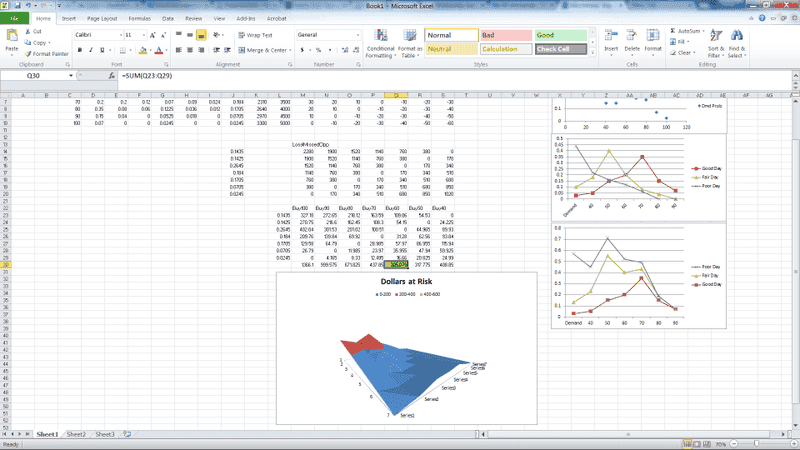mfb
Mentor
Here's a stab at 5. Buy 60? Total at risk dollars per day is lowest at @305
I would maximize the expectation value. Risk averages out over multiple days.

Ibix